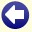## Solution to: To Know or not To Know

Let a be equal to m × n and let b be equal to m + n.

From the first remark (X: "I have no idea what your sum is, Y.") follows that a can be factorized in more than one way. If, for example, X had the number 21, which is the product of the prime numbers 3 and 7, then he would have known immediately that Y could only have got 3+7=10 as sum.

From the second remark (Y: "That's no news to me, X. I already knew you didn't know that.") follows that b cannot be written as the sum of two prime numbers. This also implies that b is not even, because each even number can be written as the sum of two prime numbers. If, for example, Y would have got the number 10, which is equal to 2+8, 3+7, 4+6, or 5+5, then Y could not have known for sure that X could not find out his sum, because X might have got the number 3×7=21 and immediately have known Y's number.

So only a limited amount of possibilities are left for the number b that Y has: 11, 17, 23, 27, 29, 35, 37, 41, 47, 51, 53, 57, 59, 65, 67, 71, 77, 79, 83, 87, 89, 93, 95, 97 (for all these numbers holds that if you subtract 2 of it, you are left with a non-prime number; all even numbers are out anyway, and all odd numbers which can be written as a prime number plus 2 are out too). There are 659 combinations of m and n with which these numbers can be combined as a sum. All these combinations have corresponding products.

As an example, the cases b=11 and b=17 have been worked out below. For convenience, we assume that m is smaller than n:

b=11
 m × n = a 2 9 18 3 8 24 4 7 28 5 6 30
b=17
 m × n = a 2 15 30 3 14 42 4 13 52 5 12 60 6 11 66 7 10 70 8 9 72

From the third remark (X: "Aha! Now I know what your sum must be, Y!") we conclude that the number a that X has, is apparently found at only one value for b. If, for example, X had the number 30, he could not find out whether Y has the number 11 or 17. As can be seen above, the value a=30 is found both at b=11 and b=17 (both 5×6 and 2×15 are equal to 30).

So, for the cases b=11 and b=17 the value of 30 for a is out. For the case b=17 the following values for a are out too:

• 42 (since 2×21=42 and 2+21=23 appears in the list of possible values for b),
• 60 (since 3×20=60 and 3+20=23 appears in the list of possible values for b),
• 66 (since 2×33=66 and 2+33=35 appears in the list of possible values for b),
• 70 (since 2×35=70 and 2+35=37 appears in the list of possible values for b),
• 72 (since 3×24=72 and 3+24=27 appears in the list of possible values for b).

Of the 659 products for all possible values of b, only the 336 products that are present once remain. For the cases b=11 and b=17 the following possibilities remain:

b=11
 m × n = a 2 9 18 3 8 24 4 7 28
b=17
 m × n = a 4 13 52

From the fourth remark (Y: "And now I also know what your product is, X!") we conclude that for the number b that Y has, there is apparently only one value of a possible. If, for example, X would have got the number 24, he could find out that Y has the number 11, but Y could not find out whether X has the number 18, 24, or 28. As can be seen above, the value b=17 gives only one possible value for a. This also turns out to be the only value for b for which this holds.

Conclusion: the numbers m and n are 4 and 13.Back to the puzzle
This website uses cookies. By further use of this website, or by clicking on 'Continue', you give permission for the use of cookies. If you want more information, look at our cookie policy.